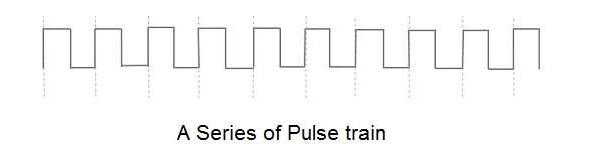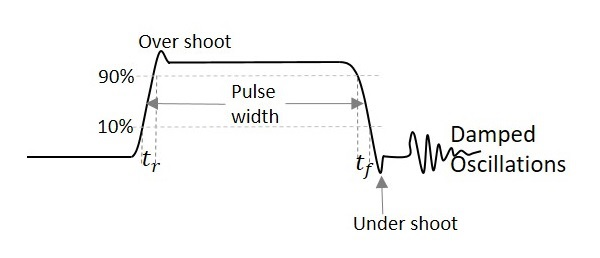# Pulse Circuits - Signal

A Signal not only carries information but it also represents the condition of the circuit. The functioning of any circuit can be studies by the signal it produces. Hence, we will start this tutorial with a brief introduction to signals.

## Electronic Signal

An electronic signal is similar to a normal signal we come across, which indicates something or which informs about something. The graphical representation of an electronic signal gives information regarding the periodical changes in the parameters such as amplitude or phase of the signal. It also provides information regarding the voltage, frequency, time period, etc.

This representation brings some shape to the information conveyed or to the signal received. Such a shape of the signal when formed according to a certain variation, can be given different names, such as sinusoidal signal, triangular signal, saw tooth signal and square wave signal etc.

These signals are mainly of two types named as Unidirectional and Bidirectional signals.

• Unidirectional Signal − The signal when flows only in one direction, which is either positive or negative, such a signal is termed as Unidirectional signal.

Example − Pulse signal.

• Bidirectional Signal − The signal when alters in both positive and negative directions crossing the zero point, such a signal is termed as a Bidirectional signal.

Example − Sinusoidal signal.

In this chapter, we are going to discuss pulse signals and their characteristic features.

## Pulse Signal

A Pulse shape is formed by a rapid or sudden transient change from a baseline value to a higher or lower level value, which returns to the same baseline value after a certain time period. Such a signal can be termed as Pulse Signal.

The following illustration shows a series of pulses.A Pulse signal is a unidirectional, non-sinusoidal signal which is similar to a square signal but it is not symmetrical like a square wave. A series of continuous pulse signals is simply called as a pulse train. A train of pulses indicate a sudden high level and a sudden low level transition from a baseline level which can be understood as ON/OFF respectively.

Hence a pulse signal indicates ON & OFF of the signal. If an electric switch is given a pulse input, it gets ON/OFF according to the pulse signal given. These switches which produce the pulse signals can be discussed later.

## Terms Related to Pulse signals

There are few terms related to pulse signals which one should know. These can be understood with the help of the following figure.From the above figure,

• Pulse width − Length of the pulse

• Period of a waveform − Measurement from any point on one cycle to the same point on next cycle

• Duty cycle − Ratio of the pulse width to the period

• Rise time − Time it takes to rise from 10% to 90% of its maximum amplitude.

• Fall time − Time signal takes to fall from 90% to 10% of its maximum amplitude.

• Overshoot − Said to be occurred when leading edge of a waveform exceeds its normal maximum value.

• Undershoot − Said to be occurred when trailing edge of a waveform exceeds its normal maximum value.

• Ringing − Both undershoot and overshoot are followed by damped oscillations known as ringing.

The damped oscillations are the signal variations that indicate the decreasing amplitude and frequency of the signal which are of no use and unwanted. These oscillations are simple disturbances known as ringing.

In the next chapter, we will explain the concept of switching in electronics done using BJTs. We had already discussed switching using diodes in our ELECTRONIC CIRCUITS tutorial. Please refer.